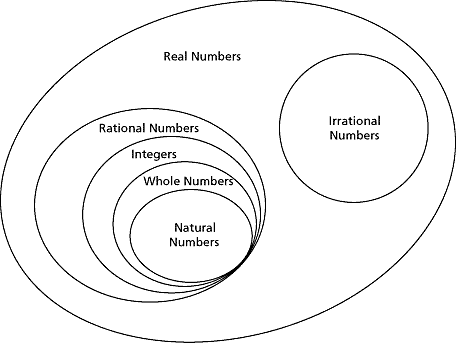1. /
2. CBSE
3. /
4. Class 07
5. /
6. Rational numbers worksheet for...

# Rational numbers worksheet for class 7### myCBSEguide App

Download the app to get CBSE Sample Papers 2023-24, NCERT Solutions (Revised), Most Important Questions, Previous Year Question Bank, Mock Tests, and Detailed Notes.

## Rational numbersworksheet for class 7 Important Topics

• Introduction to rational numbers and their need
• Positive and negative natural numbers and their representation on number line
• Rational numbers in standard form
• Representation of rational number as a decimal
• Comparison of rational numbers
• Rational numbers between two rational numbers
• Operations in rational numbers
• Word problems on rational numbers (all operations)## Some important Facts about Rational numbersworksheet for class 7

1. A number that can be expressed in the form p q , where p and q are integers and q 0, is called a rational number. The numbers 2 3, ,3 7 8 etc. are rational numbers.
2. All integers and fractions are rational numbers.
3. If the numerator and denominator of a rational number are multiplied or divided by a non-zero integer, we get a rational number which is said to be equivalent to the given rational number. For example 3 3 2 6 7 7 2 14 . So, we say 6 14 is the equivalent form of 3 7 . Also note that 6 6 2 3 14 14 2 7 .
4. Rational numbers are classified as Positive and Negative rational numbers. When the numerator and denominator, both, are positive integers, it is a positive rational number. When either the numerator or the denominator is a negative integer, it is a negative rational number. For example, 3 8 is a positive rational number whereas 8 9 is a negative rational number.
5. The number 0 is neither a positive nor a negative rational number.
6. A rational number is said to be in the standard form if its denominator is a positive integer and the numerator and denominator have no common factor other than 1. The numbers 1 2 , 3 7 etc. are in standard form.
7. There are unlimited number of rational numbers between two rational numbers.
8. Two rational numbers with the same denominator can be added by adding their numerators, keeping the denominator same. Two rational numbers with different denominators are added by first taking the LCM of the two denominators and then converting both the rational numbers to their equivalent forms having the LCM as the denominator. For example, 2 3 16 9 16 9 7 3 8 24 24 24 24 . Here, LCM of 3 and 8 is 24.

## NCERT class 7Mathematics Solved Worksheets

• Chapter 1 – Integers
• Chapter 2 – Fractions and Decimals
• Chapter 3 – Data Handling
• Chapter 4 – Simple Equations
• Chapter 5 – Lines and Angles
• Chapter 6 – Practical Geometry
• Chapter 7 – The Triangle and its Properties
• Chapter 8 – Congruence of triangles
• Chapter 9 – Comparing Quantities
• Chapter 10 – Rational numbers
• Chapter 11 – Perimeter and Area
• Chapter 12 – Algebraic Expressions
• Chapter 13 – Exponents and Powers
• Chapter 14 – Symmetry
• Chapter 15 – Visualizing Solid Shapes

## RATIONAL NUMBERS BETWEEN TWO RATIONAL NUMBERS

Reshma wanted to count the whole numbers between 3 and 10. From her earlier classes, she knew there would be exactly 6 whole numbers between 3 and 10. Similarly, she wanted to know the total number of integers between –3 and 3. The integers between –3 and 3 are –2, –1, 0, 1, 2. Thus, there are exactly 5 integers between –3 and 3. Are there any integers between –3 and –2? No, there is no integer between –3 and –2. Between two successive integers the number of integers is 0.

To download Printable worksheets for class 7 Mathematics and Science; do check myCBSEguide app or website. myCBSEguide provides sample papers with solution, test papers for chapter-wise practice, NCERT solutions, NCERT Exemplar solutions, quick revision notes for ready reference, CBSE guess papers and CBSE important question papers. Sample Paper all are made available through the best app for CBSE students and myCBSEguide website.### Test Generator

Create question paper PDF and online tests with your own name & logo in minutes.### myCBSEguide

Question Bank, Mock Tests, Exam Papers, NCERT Solutions, Sample Papers, Notes